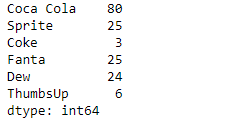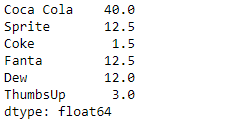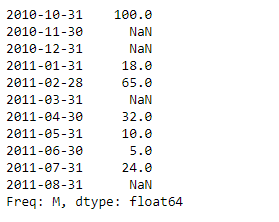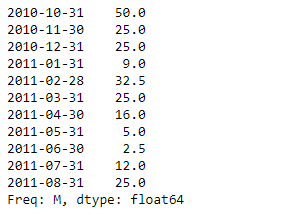# Python | Pandas Series.divide()

• Last Updated : 15 Feb, 2019

Pandas series is a One-dimensional ndarray with axis labels. The labels need not be unique but must be a hashable type. The object supports both integer- and label-based indexing and provides a host of methods for performing operations involving the index.

Pandas` Series.divide()` function performs floating division of series and other, element-wise (binary operator truediv). It is equivalent to `series / other`, but with support to substitute a fill_value for missing data in one of the inputs.

Syntax: Series.divide(other, level=None, fill_value=None, axis=0)

Parameter :
other : Series or scalar value
fill_value : Fill existing missing (NaN) values.
level : Broadcast across a level, matching Index values on the passed MultiIndex level

Returns : result : Series

Example #1: Use `Series.divide()` function to perform floating division of the given series object with a scalar.

 `# importing pandas as pd ` `import` `pandas as pd ` ` `  `# Creating the Series ` `sr ``=` `pd.Series([``80``, ``25``, ``3``, ``25``, ``24``, ``6``]) ` ` `  `# Create the Index ` `index_ ``=` `[``'Coca Cola'``, ``'Sprite'``, ``'Coke'``, ``'Fanta'``, ``'Dew'``, ``'ThumbsUp'``] ` ` `  `# set the index ` `sr.index ``=` `index_ ` ` `  `# Print the series ` `print``(sr) `

Output :Now we will use `Series.divide()` function to perform floating division of the given series object with a scalar.

 `# perform floating division ` `result ``=` `sr.divide(other ``=` `2``) ` ` `  `# Print the result ` `print``(result) `

Output :As we can see in the output, the `Series.divide()` function has successfully performed the floating division of the given series object with a scalar.

Example #2 : Use `Series.divide()` function to perform floating division of the given series object with a scalar. The given series object contains some missing values.

 `# importing pandas as pd ` `import` `pandas as pd ` ` `  `# Creating the Series ` `sr ``=` `pd.Series([``100``, ``None``, ``None``, ``18``, ``65``, ``None``, ``32``, ``10``, ``5``, ``24``, ``None``]) ` ` `  `# Create the Index ` `index_ ``=` `pd.date_range(``'2010-10-09'``, periods ``=` `11``, freq ``=``'M'``) ` ` `  `# set the index ` `sr.index ``=` `index_ ` ` `  `# Print the series ` `print``(sr) `

Output :Now we will use `Series.divide()` function to perform floating division of the given series object with a scalar. We are going to fill 50 at the place of all the missing values.

 `# perform floating division ` `# fill 50 at the place of missing values ` `result ``=` `sr.divide(other ``=` `2``, fill_value ``=` `50``) ` ` `  `# Print the result ` `print``(result) `

Output :As we can see in the output, the `Series.divide()` function has successfully performed the floating division of the given series object with a scalar.

My Personal Notes arrow_drop_up
Recommended Articles
Page :